Trial ends in
JoVE Science Education
Mechanical Engineering

A subscription to JoVE is required to view this content.
You will only be able to see the first 20 seconds.

Measuring Turbulent Flows
##### Science Education (Mechanical Engineering)
Hot Wire Anemometry
##### Science Education (Mechanical Engineering)
Introduction to Refrigeration

# Measuring Turbulent Flows

### Overview

Source: Ricardo Mejia-Alvarez and Hussam Hikmat Jabbar, Department of Mechanical Engineering, Michigan State University, East Lansing, MI

Turbulent flows exhibit very high frequency fluctuations that require instruments with high time-resolution for their appropriate characterization. Hot-wire anemometers have a short enough time-response to fulfill this requirement. The purpose of this experiment is to demonstrate the use of hot-wire anemometry to characterize a turbulent jet.

In this experiment, a previously calibrated hot-wire probe will be used to obtain velocity measurements at different positions within the jet. Finally, we will demonstrate a basic statistical analysis of the data to characterize the turbulent field.

### Principles

A description of a turbulent flow

A turbulent flow can be evidenced by highly random fluctuations in flow variables such as velocity, pressure, and vorticity. Figure 1 represents a typical velocity signal obtained by measuring velocity at a fixed point in a turbulent flow. The fluctuations in this signal are not random noise, but the result of non-linear interactions between coherent motions within the flow field. A classical description of turbulent flow, involves the determination of the average value of flow variables and their corresponding fluctuations as time progresses. To this end, we use the definition for the average of a function to determine the average of a velocity measurement: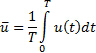(1)

Here,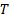is the size of the integration domain, which will be a time interval in the present measurements. As hinted by equation (1), we will use an overbar to denote the average of a variable. Given that a digital acquisition of a signal is discrete, the integral in equation (1) should be solved numerically, using either the trapezoidal or the Simpson's rule . The fluctuations of a time-dependent variable like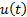can then be calculated as follows: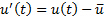(2)

As seen in this equation, fluctuation fields are denoted by a prime symbol. By applying equation (1) to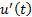, we can easily determine that the average of a fluctuation field is zero: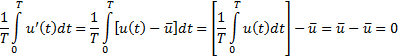(3)

Hence, a more appropriate statistical descriptor for the fluctuation field is the root mean square of the fluctuations: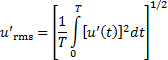(4)

This statistical descriptor is in fact a very common measure of the turbulence intensity. The current experiment will be based on determining the average velocity and turbulence intensity of a turbulent field.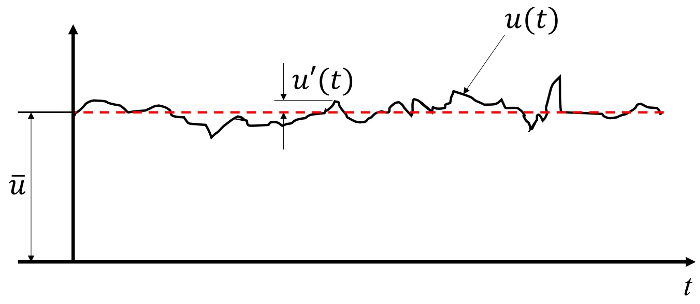Figure 1. Typical signal of velocity of a turbulent flow as recovered by a hot-wire anemometer. The raw signal,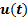, can be decomposed in a fluctuation field,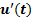, superimposed on the average value of velocity,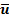.

Experimental setup

As shown in Figure 2(A) the facility is basically a plenum that gets pressurized by a centrifugal fan. Figure 2(B) shows that there is a slit on the opposite side of the plenum that issues a planar jet. As shown in Figure 2(C), a traversing system holds the hot-wire anemometer at prescribed locations in the planar jet. This traversing system will be used to determine the velocity at different positions of interest in the jet. The schematic of Figure 3 shows a representative location at which anemometry will be carried out in order to characterize the turbulent field in the planar jet.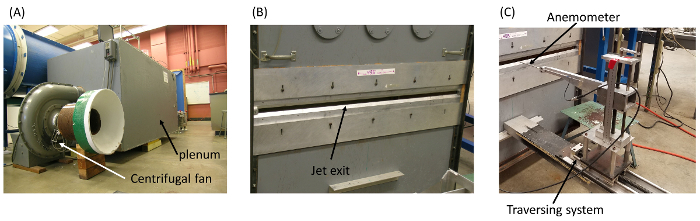Figure 2. Experimental setup. (A): flow facility; the plenum is pressurized by means of a centrifugal fan. (B): slit for issuing the planar jet. (C): traversing system to change the position of the anemometer along the jet. Please click here to view a larger version of this figure.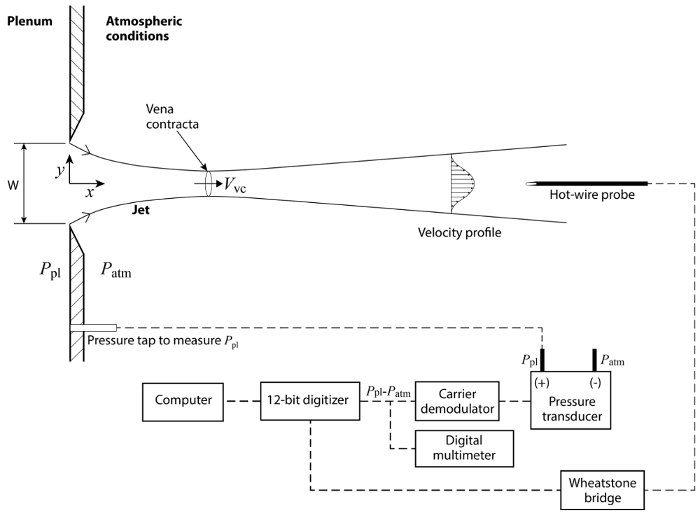Figure 3. Schematic of the planar jet showing: the vena contracta, the velocity distribution at a given downstream position, and the diagram of connections. Please click here to view a larger version of this figure.

### Procedure

1. Measure the width of the slit, W, and record this value in table 1.
2. Set the hot-wire anemometer at a distance from the exit equal to x = 1.5W along the centerline. Record this streamwise position in table 2. The centerline is the origin of the spanwise coordinate (y = 0).
3. Start the data acquisition program for traversing the jet. Set the sample rate at 500 Hz for a total of 5000 samples (i.e. 10s of data).
4. Record the current spanwise position of the hot-wire in table 3.
5. Acquire data.
6. The data acquisition system will calculate the average velocity and turbulence intensity of that dataset using equations (1) and (4).
7. Record those two values in table 3.
8. Move the hotwire to the next (positive) spanwise position (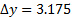mm).
9. Repeat steps 5 to 8 until there is not any noticeable change on both the average velocity and the turbulence intensity.
10. Move the hot-wire back to the centerline.
11. Move the hotwire to the next (negative) spanwise position (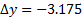mm).
12. Acquire data.
13. The data acquisition system will calculate the average velocity and turbulence intensity of that dataset using equations (1) and (4).
14. Record those two values in table 3.
15. Repeat steps 11 to 14 until there is not any noticeable change on both the average velocity and the turbulence intensity.
16. Move the hot-wire back to the centerline of the jet.
17. Move the hot-wire along the centerline of the jet in the downstream direction to a new position (e.g. x = 3W).
18. Repeat steps 4 to 17 for as many streamwise positions as wanted (e.g. x = 1.5W, 3W, 6W, 9W).

Table 1 . Basic parameters for experimental study.

 Parameter Value Slit width (W) 19.05 mm Air density (r) 1.2 kg/m3 Transducer calibration constant (m_p) 76.75 Pa/V Calibration constant A 5.40369 V2 Calibration constant B 2.30234 V2(m/s)-0.65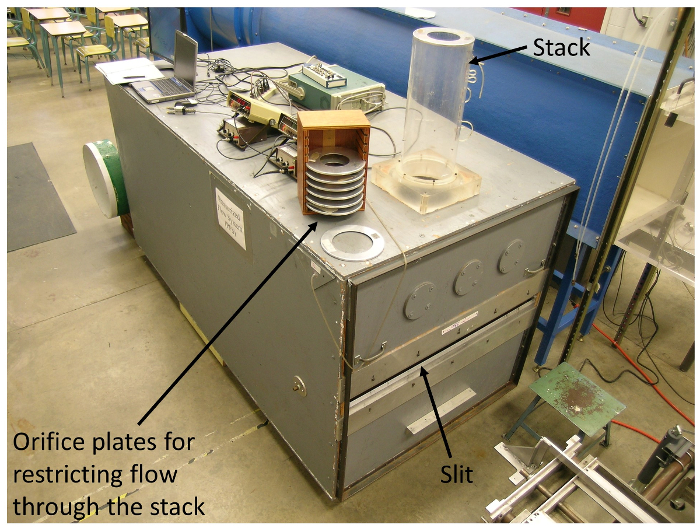Figure 4. Flow control in the flow system. The stack on top of the plenum serves the purpose of diverting flow from the jet slit allowing to control the jet ' s exit velocity. Please click here to view a larger version of this figure.

Turbulent flows play an important role in a wide variety of engineered and naturally-occurring systems. As a result, it is often necessary to perform measurements within the system in order to characterize the flow. Turbulent flows exhibit very high frequency fluctuations so any instrument that is used to measure and characterize turbulence must have a high enough time resolution to resolve these changes. Hot wire anemometers are often used for these measurements because they are small, robust, and fast enough to yield useful results. This video will illustrate how to use a calibrated hot wire anemometer probe to obtain velocity and turbulence measurements at different positions within a free jet and then perform a basic statistical analysis of the data to characterize the turbulent field.

A turbulent flow can be evidenced by high random fluctuations in flow variables such as velocity, pressure, and vorticity. These fluctuations are the result of nonlinear interactions between coherent motions within the flow field so the high frequency oscillations seen in measurements of turbulence are from real physical effects and not the result of random electronic noise. A classical description of turbulent flow involves the determination of the average value of flow variables and their corresponding fluctuations with time. For example, the average velocity, denoted by an over bar, is found by integrating the instantaneous velocity over the measurement time and scaling by the size of the integration domain. In the case of discrete measurements such as those from digital acquisition systems, the integral must be solved numerically. Once the average velocity has been found, it can be subtracted from the original signal to yield the time-dependent fluctuation and velocity denoted by the prime. From these definitions, it is easy to show that the average of a fluctuation field is zero. As a result, a more appropriate statistical descriptor for the fluctuation field is needed. A very common measure is the Root Mean Square or RMS of the fluctuations. This metric is similar to the average, except that the variable is squared before integrating and the square root of the result is taken. The turbulence intensity is given by the RMS of the velocity and this measurement will be demonstrated on a free jet in the next section. The average velocity of a free jet has an initially flat-top profile that smooths out as the jet propagates due to entrainment of surrounding air into the jet. This entrainment also causes the linear momentum of the jet to spread span-wise as the jet flows downstream resulting in widening of the jet as it propagates. The region of interaction between the jet and surrounding air is called the mixing layer and this region grows towards the center line as the jet moves downstream. This leaves a region inside the jet known as the potential core that is delimited in the stream-wise direction by the jet exit and the point at which the mixing layer reaches the center line. The potential core is then a region that has not been affected by interactions with the surrounding environment. On the center line, the potential core extends downstream to about four times the width of the jet exit. Now that you are familiar with the basics of turbulence measurements, let's look at how this can be used to characterize a free jet.

Before you begin setting up, familiarize yourself with the layout and safety procedures of the facility. This experiment will be performed on the same flow system that was used for the hot wire anemometer calibration and the data acquisition system should be setup in the same manner. In the data acquisition software, set the sampling rate to 500 Hertz and the total samples to 5,000. Update the constants n, A, and B to match the values determined from the calibration. Now set up the flow facility. Use a calibrated spacer to set the slit width to 19.05 millimeters or three-quarters of an inch and then translate the hot wire anemometer to the vena contracta of the jet 1.5 times the slit width away from the exit. Starting with the anemometer above the slit, lower the height until the signal on the oscilloscope reaches a minimum fluctuation. Record this vertical position which corresponds to the center line of the jet. Now translate the anemometer back up until the signal fluctuation is a maximum and this position corresponds to the upper shear layer of the jet. Insert the blank orifice plate into the stack so that the flow velocity will be maximized and then turn on the flow facility. Once steady flow is established, use the data acquisition system to measure the average velocity and turbulence intensity at this point in the jet and record these values. Now move the anemometer span-wise down by two millimeters and measure the average velocity and turbulence intensity again. Continue lowering the anemometer by two millimeter increments and taking measurements until there is no noticeable change in both measurements. After recording the final height, translate the anemometer down until it is below the center line by the same distance. Resume taking measurements and translating up until the anemometer is back at the center line. When you are finished, translate the anemometer downstream until it is three times the slit width from the jet exit. Take measurements of the jet profile at this new stream-wise position following the same procedure you used in the first location. Repeat your measurements of the jet profile at six and nine times the slit width from the jet exit. After you've completed the measurements, shut down the flow facility.

Take a look at your data. At each stream-wise position, you have measurements of the average velocity and the turbulence intensity taken at a series of span-wise points. First plot the average velocity as a function of the span-wise position. Scale the values by the center line value and find the points where the curve intersects the 50% threshold, interpolating as necessary. These points define the jet width Delta at this stream-wise position. Calculate the width by taking the difference. In this case, the width is about 21.5 millimeters. Now compare the average center line velocity and jet width at the different stream line positions. The center line velocity remains basically unchanged up to about four times the slit width from the exit due to the potential core, but decreases beyond this distance. The increase in jet width with distance is indicative of the span-wise spread of the linear momentum of the jet as the surrounding air is entrained. Now plot the turbulence intensity as a function of the span-wise position. Since mixing happens at the boundary between the jet and the surrounding environment, turbulence intensity peaks away from the center line.

Turbulent flow is ubiquitous in scientific and engineering applications. For its assessment in engineering applications such as ventilation, heating, and air conditioning, it is common to use portable hot wire probes that are introduced to the ducting and traverse radially to obtain the velocity profiles. This information is then used by the engineer to either balance a newly-installed flow system to ensure its proper operation or to troubleshoot a malfunctioning system and solve any problem that hinders its operation. When a new terrestrial, aerial, or marine vehicle or structure is designed to stand the forces of turbulent flows, it is necessary to test its performance under realistic flow conditions in a wind or water tunnel. To simulate turbulence conditions that occur in the atmosphere or ocean, the incoming flow can be disturbed with active or passive grids that will introduce significant fluctuations in the flow. Then the vehicle or structure under study can be mounted in the test section of the wind or water tunnel to measure how it copes with the loads introduced by the turbulent flow. These measurements can be made directly with aerodynamic balances that measure the resulting drag and lift forces. Also, the velocity around the model tested in the tunnel might give important information regarding performance. This characterization is typically made with hot wire anemometers in wind tunnels.

You've just watched Jove's introduction to measuring turbulent flows. You should now understand how to deploy hot wire anemometers to measure and assess flow profiles and turbulence intensity. Thanks for watching.

### Results

Figure 5 shows the distribution of average velocity across the jet at the downstream position x = 3W. And Figure 6 shows the distribution of turbulence intensity across the jet at the same downstream position. Table 3 has the results for the local values of average velocity and turbulence intensity at the streamwise position x = 3W. The last column of this table is the ratio between the local velocity and the centerline velocity. This ratio is used to determine the jet width,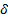, which is defined as the distance between the two positions at which the local velocity is 50% of the centerline velocity. Note from table 2 that these two positions are somewhere in the intervals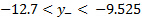and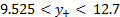. Their exact locations are determined using linear interpolation, and are determined to be: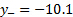mm and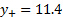mm, for a jet thickness of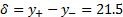mm.

The results for four different experiments are compared in table 2. This table shows how the centerline velocity of the jet,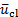, remains basically unchanged for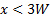, but decreases with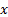for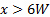. This effect is the result of the presence of the potential core for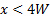, and its disappearance for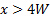. The potential core is the region inside the jet that has not been affected by the interaction between the environment and the jet. The region of interaction is called the mixing layer, and it grows toward the centerline and away from the jet as the jet moves downstream. This growth is due to entrainment of surrounding air into the jet. Due to this entrainment effect, the linear momentum of the jet spreads in the spanwise direction, causing its width to increase with. This effect is evidenced by the results foron table 2. Due to the fact that mixing happens at the boundary between the jet and the surrounding environment, the turbulence intensity peaks (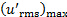) away from the centerline, at spanwise positions defined by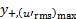and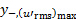. For simplicity, Table 2 only shows the values for the peak of turbulence intensity at the positive side of the jet.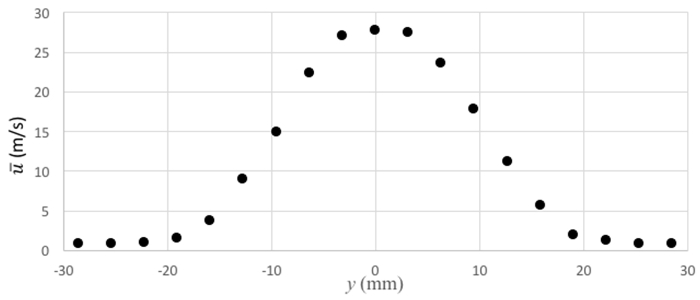Figure 5. Representative results. Velocity distribution at x = 3W.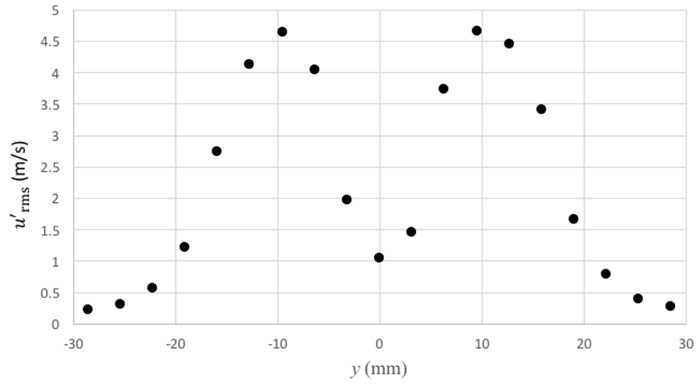Figure 6. Representative results. Turbulence intensity distribution at x = 3W.

Table 2. Representative results. Different statistical descriptors for the planar jet at x = 1.5W, 3W, 6W, and 9W.

 x/W u ̅_cl (m/s) δ (mm) (u′_rms )_max (m/s) y_(+,(u′_rms )_max ) 1.5 27.677 19.37 4.919 0.9525 3.0 27.706 21.50 4.653 0.9525 6.0 24.783 28.18 4.609 0.9525 9.0 20.470 39.68 4.513 1.2700

Table 3. Representative results. Measurements of velocity and turbulence intensity at x = 3W.

 y (mm) u ̅ (m/s) u′_rms (m/s) u ̅∕u ̅_cl -28.575 0.762 0.213 0.028 -25.400 0.783 0.311 0.028 -22.225 0.949 0.554 0.034 -19.050 1.461 1.218 0.053 -15.875 3.751 2.727 0.135 -12.700 8.941 4.114 0.323 -9.525 14.919 4.633 0.538 -6.350 22.383 4.043 0.808 -3.175 26.952 1.958 0.973 0.000 27.706 1.039 1.000 3.175 27.416 1.455 0.990 6.350 23.573 3.730 0.851 9.525 17.748 4.653 0.641 12.700 11.175 4.443 0.403 15.875 5.583 3.399 0.202 19.050 1.943 1.663 0.070 22.225 1.159 0.785 0.042 25.400 0.850 0.383 0.031 28.575 0.877 0.271 0.032

### Applications and SummaryX